# Xbar R Charts with SigmaXL

### What is a Xbar R Chart?

The Xbar R chart is a control chart for continuous data with a constant subgroup size between two and ten.

• The Xbar chart plots the average of a subgroup as a data point.
• The R chart plots the difference between the highest and lowest values within a subgroup as a data point.

The Xbar chart monitors the process mean and the R chart monitors the variation within subgroups. The Xbar is valid only if the R chart is in control.
The underlying distribution of the Xbar-R chart is normal distribution.

### Xbar Chart Equations

Xbar chart

Data Point: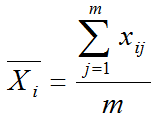Center Line: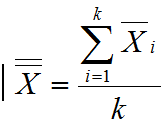Control Limits: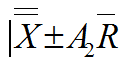Where:

• m is the subgroup size
• k is the number of subgroups

A2 is a constant depending on the subgroup size.

### R Chart Equations

R chart (Rage Chart)
Data Point:

Center Line: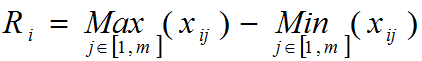Upper Control Limit: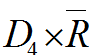Lower Control Limit: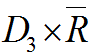Where:

• m is the subgroup size and k is the number of subgroups
• D3 and D4 are constants depending on the subgroup size

### Use SigmaXL to Plot Xbar-R Charts

Data File: “Xbar-R” tab in “Sample Data.xlsx”

Steps to plot Xbar-R charts in SigmaXL:

1. Select the entire range of the data
2. Click SigmaXL -> Control Charts -> X-Bar & R
3. A new window named “X-Bar & R” appears with the selected range automatically populated into the box below “Please select your data”.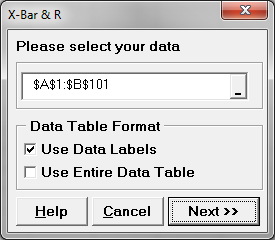4. Click “Next>>”
5. A new window named “X-Bar and Range Chart” pops up.
6. Select the “Measurement” as the “Numeric Data Variables (Y)”
Select the “Subgroup ID” as the “Subgroup Column or Size”
7. Check the checkbox of “Tests for Special Causes”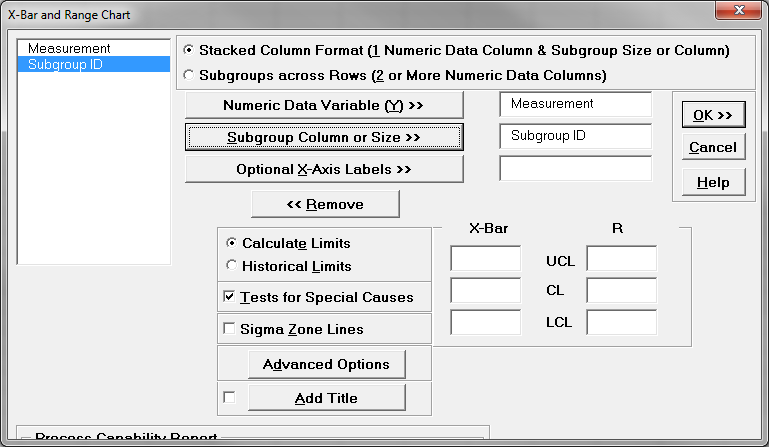8. Click “OK>>”
9. The Xbar-R charts appear in the newly generated tab “Indiv & MR Charts (1)”.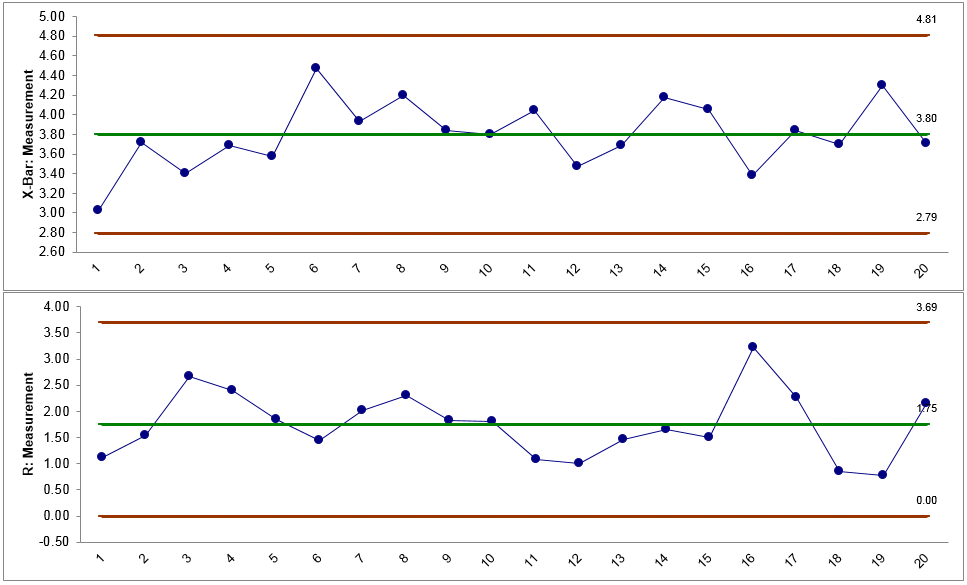#### Xbar-R Charts Diagnosis

Model summary: Since the R chart is in control, the Xbar chart is valid and no points on the Xbar chart are out of control. In both charts, there are not any data points failing any tests for special causes (i.e., all the data points fall between the control limits and spread around the center line with a random pattern). We conclude that the process is in control.